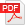Full presentation

# Solving elliptic PDE in Scilab with the Feynman-Kac formula

### Giovanni Conforti Berlin Mathematical School

In this talk we describe and implement in Scilab a stochastic numerical algorithm to solve elliptic PDEs with special focus on the heat equation. The algorithm is based on the celebrated Feynman-Kac representation formula. This formula reduces the problem of solving the heat equation to the computation of expected values of functionals of the sample path of the Brownian motion.

Thanks to the Law of Large Numbers, Monte Carlo methods provide a robust tool to approximate expectations. The aim of the talk is twofolded. Beside bringing the attention to such approximation schemes, by discussing both theoretical aspects and simulations in comparison with other PDE solvers, we would like to introduce the main tools that Scilab offers to tackle problems arising from stochastic modelling.